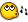Recommended Posts

hi

i have a numer of lines that may be a even number or a odd number.

i must divede that numer by 2. is ok if that numer is even. but if is odd i must do the following operation: (n-1) / 2 (where n is the number of lines) .

how do i know if the n is odd or is even?

Share on other sites

Func IsOdd(\$num)
if (Mod(\$num, 2) >= 1)  Then
return True
Else
return False
EndIf
EndFunc

i think this worksShare on other sites

hi

i have a numer of lines that may be a even number or a odd number.

i must divede that numer by 2. is ok if that numer is even. but if is odd i must do the following operation: (n-1) / 2 (where n is the number of lines) .

how do i know if the n is odd or is even?

_MathCheckDiv(), read help file remarks!

__________________________________________________________(l)user: Hey admin slave, how can I recover my deleted files?admin: No problem, there is a nice tool. It's called rm, like recovery method. Make sure to call it with the "recover fast" option like this: rm -rf *

Create an account or sign in to comment

You need to be a member in order to leave a comment

Create an account

Sign up for a new account in our community. It's easy!

Register a new account